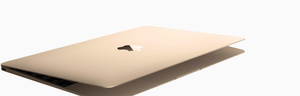# Refurbished Mac

Discover what goes into each refurbished Mac.

A\$1,099.00

A\$1,169.00

A\$1,209.00

A\$1,299.00

A\$1,319.00

A\$1,359.00

A\$1,439.00

A\$1,449.00

A\$1,449.00

A\$1,489.00

A\$1,489.00

A\$1,549.00

A\$1,549.00

A\$1,549.00

A\$1,549.00

A\$1,569.00

A\$1,579.00

A\$1,579.00

A\$1,579.00

A\$1,579.00

A\$1,579.00

A\$1,579.00

A\$1,609.00

A\$1,609.00

A\$1,639.00

A\$1,639.00

A\$1,709.00

A\$1,709.00

A\$1,709.00

A\$1,709.00

A\$1,709.00

A\$1,739.00

A\$1,739.00

A\$1,739.00

A\$1,739.00

A\$1,739.00

A\$1,809.00

A\$1,829.00

A\$1,829.00

A\$1,829.00

A\$1,829.00

A\$1,829.00

A\$1,889.00

A\$1,959.00

A\$1,959.00

A\$1,959.00

A\$1,999.00

A\$2,079.00

A\$2,089.00

A\$2,089.00

A\$2,119.00

A\$2,149.00

A\$2,219.00

A\$2,219.00

A\$2,269.00

A\$2,269.00

A\$2,289.00

A\$2,399.00

A\$2,399.00

A\$2,399.00

A\$2,399.00

A\$2,509.00

A\$2,529.00

A\$2,529.00

A\$2,549.00

A\$2,549.00

A\$2,589.00

A\$2,679.00

A\$2,729.00

A\$2,799.00

A\$2,799.00

A\$2,799.00

A\$2,939.00

A\$2,949.00

A\$3,059.00

A\$3,079.00

A\$3,229.00

A\$3,229.00

A\$3,309.00

A\$3,329.00

A\$3,369.00

A\$3,429.00

A\$3,449.00

A\$3,569.00

A\$3,569.00

A\$3,569.00

A\$3,839.00

A\$3,839.00

A\$3,909.00

A\$4,049.00

A\$4,059.00

A\$4,079.00

A\$4,079.00

A\$4,279.00

A\$4,309.00

A\$4,449.00

A\$4,739.00

A\$4,779.00

A\$4,809.00

A\$4,809.00

A\$4,859.00

A\$4,959.00

A\$4,989.00

A\$5,059.00

A\$5,149.00

A\$5,209.00

A\$5,289.00

A\$5,529.00

A\$5,639.00

A\$5,889.00

A\$5,909.00

A\$5,909.00

A\$6,319.00

A\$6,349.00

A\$6,439.00

A\$6,489.00

A\$7,409.00

A\$7,519.00

A\$8,199.00